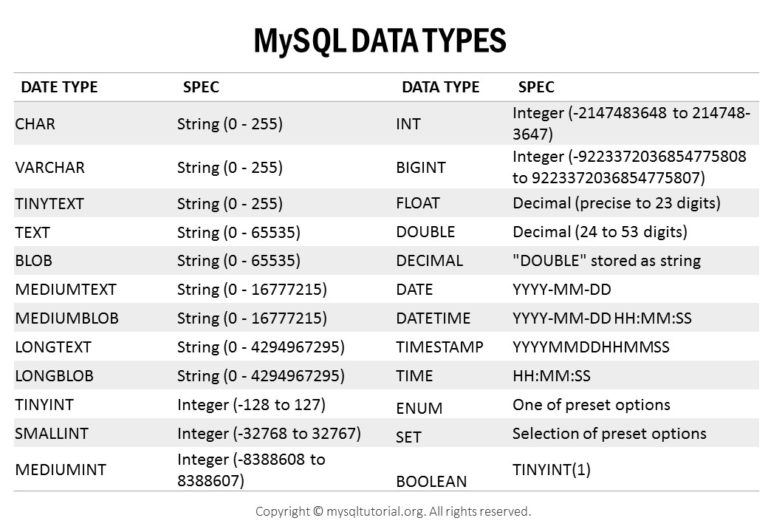#### SQL - Data Types

A database table contains multiple columns with specific data types such as numeric or string. MySQL provides more data types other than just numeric or string. Each data type in MySQL can be determined by the following characteristics:
• The kind of values it represents.
• The space that takes up and whether the values is a fixed-length or variable length.
• The values of the data type can be indexed or not.
• How MySQL compares the values of a specific data type.• Exact Numeric Data Types
• Exact (also known as fixed-point) - Exact numeric values have a fixed number of digits to the left of the decimal point and a fixed number of digits to the right (the scale). The total number of digits on both sides of the decimal are the precision. A special subset of exact numeric types with a scale of 0 is called integer.
Various Data Types: bigint, int, smallint, tinyint, bit, decimal, numeric, money, smallmoney.

• Approximate Numeric Data Types
• Approximate (also known as floating-point) - Approximate numeric values that have a fixed precision (number of digits) but a floating decimal point.
Various Data Types: float, real.

• Date and Time Data Types
• Datetime values include calendar and clock values (Date, Time, Timestamp) and intervals.
The datetime types are:

• Date - Calendar date with year, month and day.
• Time - Clock time with hour, minute, second and fraction of second, plus a timezone component (adjustment in hours, minutes).
• Timestamp - Combination calendar date and clock time with year, month, day, hour, minute, second and fraction of second, plus a timezone component (adjustment in hours, minutes).
• Interval - Intervals represent time and date intervals. They are signed. An interval value can contain a subset of the interval fields, for example - hour to minute, year, day to second.
Interval types are subdivided into:
• year-month intervals - may contain years, months or combination years/months value.
• day-time intervals - days, hours, minutes, seconds, fractions of second.

• Character Strings Data Types
• Character (String) - Fixed or variable length character strings.
The data types are: char, varchar, varchar(max), text.

• Unicode Character Strings Data Types
• Data types in Unicode Character Strings are: nchar, nvarchar, nvarchar(max), ntext.

• Binary Data Types
• Data types in Binary Data Types are: binary, varbinary, varbinary(max), image.